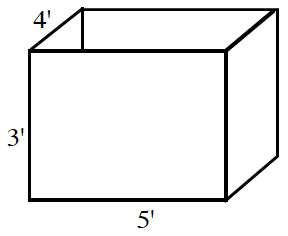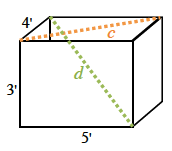### Home > CCA2 > Chapter 7 > Lesson 7.1.4 > Problem7-69

7-69.A $5' × 4' × 3'$ box is made for the purpose of storage. What is the longest pole that can fit inside the box?

Find the distance between two opposite corners of the box.

Draw a right triangle on the base of the box.
Find the measure of the hypotenuse.

$4^2 + 5^2 = c^2$Now draw a right triangle from the bottom corner to the top of the box.
Find the measure of that hypotenuse.

$c^2 + 3^2 = d^2$

$7.07'$